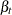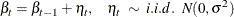### RANDOMREG Statement

RANDOMREG regressors </ options > ;

The RANDOMREG statement is used to specify regressors with time-varying regression coefficients. Each regression coefficient—say,— is assumed to evolve as a random walk:Of course, if the random walk disturbance varianceis zero, then the regression coefficient is not time varying, and it reduces to the standard regression setting. There can be multiple RANDOMREG statements, and each statement can contain one or more regressors. The regressors in a given RANDOMREG statement form a group that is assumed to share the same disturbance variance parameter. The random walks associated with different regressors are assumed to be independent. For an example of using this statement see Example 35.4. See the section Reporting Parameter Estimates for Random Regressors for additional information about the way parameter estimates are reported for this type of regressors.

NOEST

fixes the value ofto the value specified in the VARIANCE= option.

PLOT=FILTER
PLOT=SMOOTH
PLOT=( <FILTER> <SMOOTH> )

requests plotting of filtered or smoothed estimate of the time-varying regression coefficient.

PRINT=FILTER
PRINT=SMOOTH
PRINT=( <FILTER> <SMOOTH> )

requests printing of the filtered or smoothed estimate of the time-varying regression coefficient.

VARIANCE=value

specifies an initial value forduring the parameter estimation process. Any nonnegative value, including zero, is an acceptable starting value.